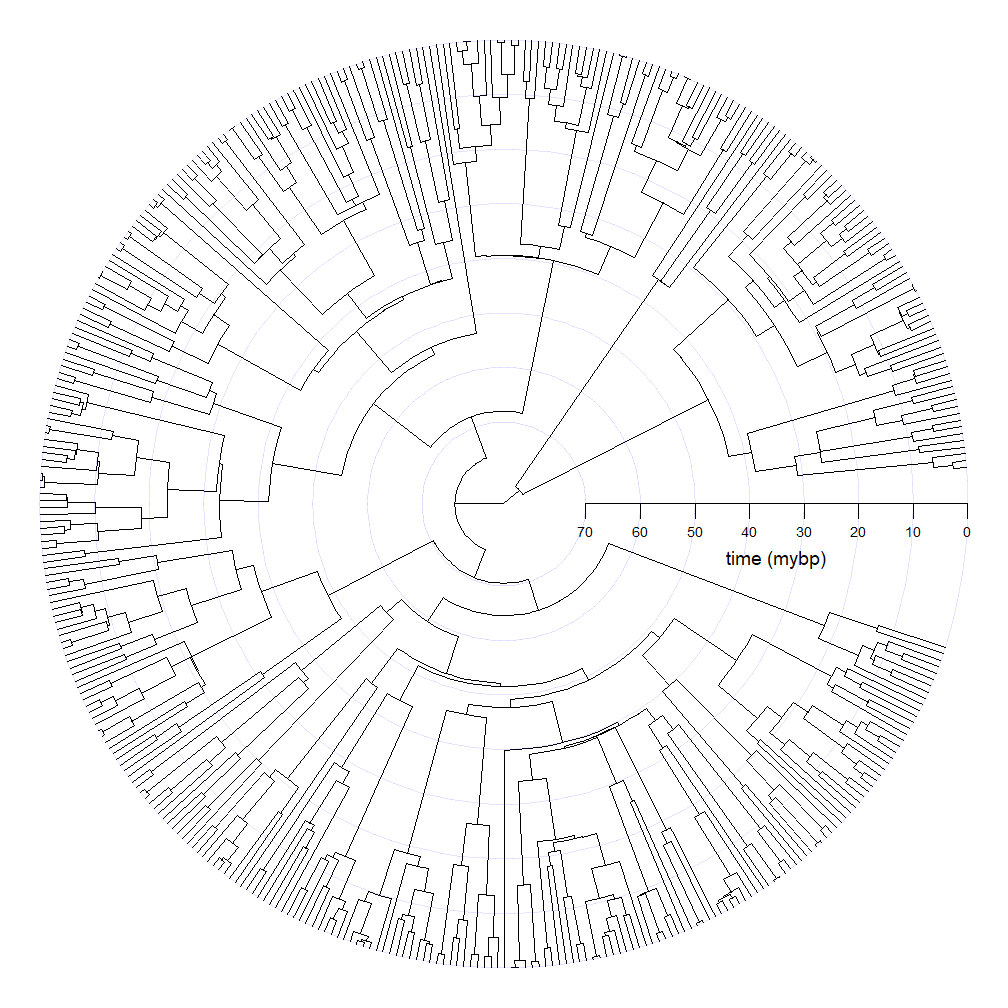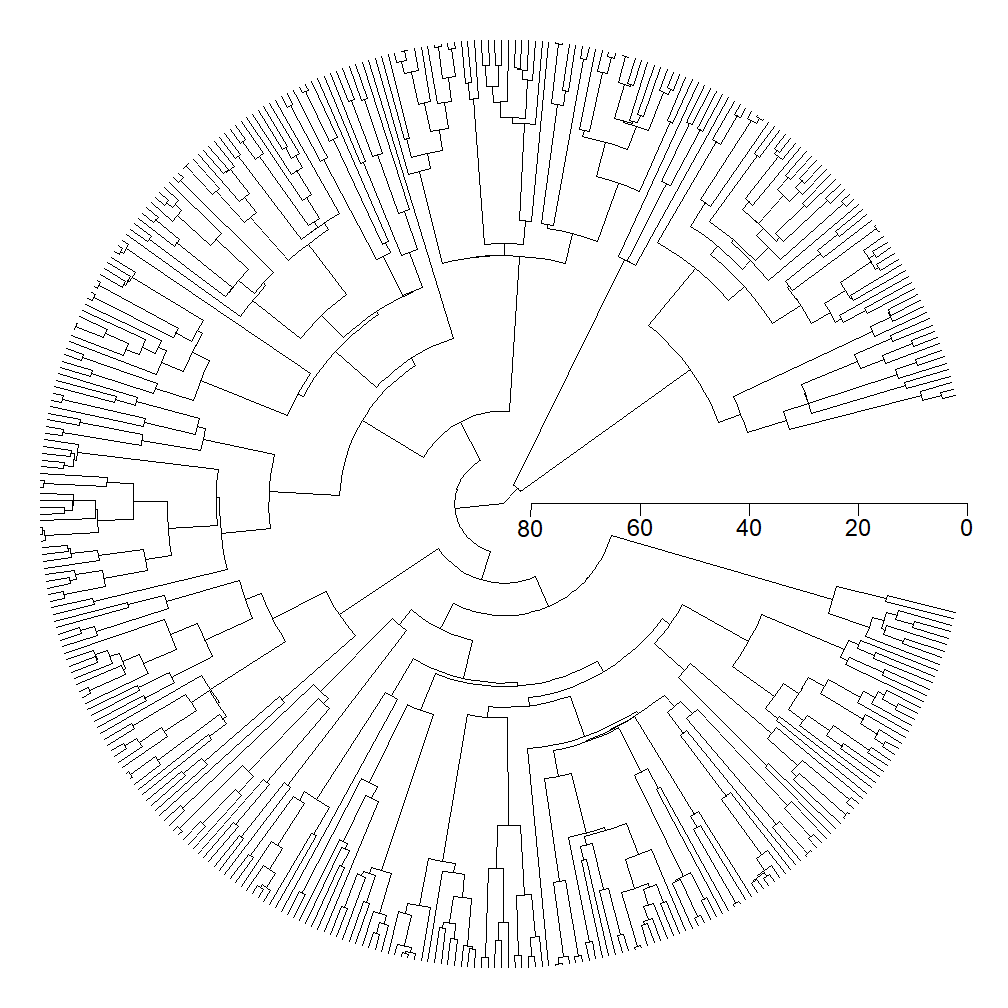## Sunday, February 4, 2018

### Another technique for including a time axis in a circular fan-style tree

In a prior post I showed how to add a time axis to a plotted fan tree using the `plotTree` / `plot.simmap` argument `part`.

Though this works well, our open angle is between `part` × 360o and 360o, rather than centered around 0o / 3600. To do this, we should ignore the argument `part` & instead combine the poorly documented arguments `tips` and `maxY`. These arguments exist to allow the user to supply a custom tip height spacing/order for a square phylogram; however, we used in a fan-style tree the effect is to transform these values into the circular space.

Here's what I mean:

``````library(phytools)
library(plotrix)
tree
``````
``````##
## Phylogenetic tree with 400 tips and 399 internal nodes.
##
## Tip labels:
##  t307, t308, t181, t128, t129, t110, ...
##
## Rooted; includes branch lengths.
``````
``````plotTree(tree,type="fan",tips=seq(5,380,by=375/399),maxY=400,
ftype="off",lwd=1)
T<-max(nodeHeights(tree))
tick.spacing<-10
min.tick<-10
obj<-axis(1,pos=0,at=seq(T,min.tick,by=-tick.spacing),cex.axis=0.5,
labels=FALSE)

for(i in 1:length(obj)){
a1<-0
a2<-2*pi
col=make.transparent("blue",0.1))
}

axis(1,pos=0,at=seq(T,min.tick,by=-tick.spacing),cex.axis=0.5,
labels=FALSE)
text(obj,rep(-5,length(obj)),T-obj,cex=0.6)
text(mean(obj),-10,"time (mybp)",cex=0.8)
``````Neat.

Note that in theory we could do this with the ape function `axisPhylo`, although this function seems to be somewhat unpredictable as to whether or not it works as expected. For instance:

``````plotTree(tree,type="fan",tips=seq(15,385,by=370/399),maxY=400,
ftype="off",lwd=1)
axisPhylo()
``````